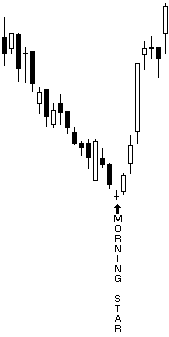# MorningStar

 Description The Morning Star pattern is the converse of the Evening Star pattern. The Morning Star is a bottom reversal pattern, so bearish trend confirmation is important.   The Morning Star is a three candlestick pattern:Ideally, the first, white bar is has a long black body. The second candlestick gaps down and has a small real body--ideally, no real body (Doji). The third candlestick has a white body that penetrates well into the first day's black real body. If the third candlestick gaps up, an Abandoned Baby pattern (Island Bottom) emerges. Formula MorningStar(INPUT, Stringency=8, LRRange=10, LRType=3, SmallForm=10)=begin   retval = NONUM   targetBar = 0   pnt0 = targetBar   pnt1 = targetBar + 1   pnt2 = targetBar + 2   ppClose = GetPrice(\$1, BATE_CLOSE, pnt2, 0)   ppLow = GetPrice(\$1, BATE_LOW, pnt2, 0)   pOpen = GetPrice(\$1, BATE_OPEN, pnt1, 0)   pClose = GetPrice(\$1, BATE_CLOSE, pnt1, 0)   pHigh = GetPrice(\$1, BATE_HIGH, pnt1, 0)   cOpen = GetPrice(\$1, BATE_OPEN, targetBar, 0)     if WhiteBody(\$1, pnt0) == TRUE AND BlackBody(\$1, pnt2) == TRUE then begin     if Stringency >= 8 then retval = '8'|clr_green|below|ftiny|arrow|vertical     if pOpen <= ppClose then begin       if Stringency >= 7 then retval = '7'|clr_green|below|ftiny|arrow|vertical       if cOpen > pClose then begin         if Stringency >= 6 then retval = '6'|clr_green|below|ftiny|arrow|vertical         if SmallRealBody(\$1, pnt1, SmallForm) then begin           if Stringency >= 5 then retval = '5'|clr_green|below|ftiny|arrow|vertical           if cOpen > pHigh then begin             if Stringency >= 4 then retval = '4'|clr_green|below|ftiny|arrow|vertical             if LongBlackForm(\$1, pnt2) OR DojiForm(\$1, pnt1) OR LongWhiteForm(\$1, pnt1) then begin               if Stringency >= 3 then retval = '3'|clr_green|below|ftiny|arrow|vertical               if LongWhiteForm(\$1, pnt1) then begin                 if Stringency >= 2 then retval = '2'|clr_green|below|ftiny|arrow|vertical                 if Bearish(\$1, pnt2, LRRange, LRType) == TRUE then begin                   retval = 'Morning Star'|clr_black|below|ftiny|arrow|vertical                 end               end             end           end         end       end     end   end   retval end Parameters INPUT The INPUT directive makes this formula available as a Formula Overlay. INPUT refers to the instrument in the chart.   Stringency The Stringency argument is used by nearly all candlestick overlays. It controls how many tests must pass in the evaluation of a Morning Star. The lower the number, the more strict the interpretation.   The Stringency argument is an overlay parameter. You can adjust it using the Parameters dialog. The default is 8.   LRRange The MorningStar overlay uses the LR() function with either a continuous or quadratic calculation to determine how the market is trending. The LRRange (Linear Regression Range) parameter is the number of points in the Linear Regression calculation. The default is 10.   LRType The LRType parameter has two possible settings: 2 and 3, or continuous linear regression and quadratic linear regression, respectively. The default is 3, or quadratic.   SmallForm The SmallForm parameter represents a percentage. This parameter serves as the basis for determining whether a candlestick is Doji or near Doji. The smaller the value assigned to SmallForm the more stringent the test. The default is 15, or fifteen percent. Return Value The return value is a string plot and varies depending on the value of the Stringency parameter.  The lower the number, the stronger the pattern.   Bullish indicators are rendered in green below the candlestick.   The string plot for a fully qualified Morning Star is "Morning Star" rendered in black below the candlestick. ExamplesComments NA